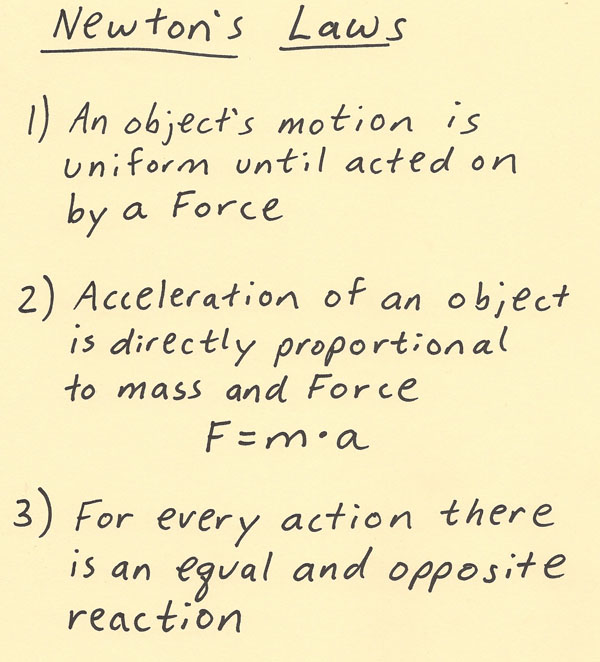[--MAIN HOME--] [--ALL HABYHINTS--] [--FACEBOOK PAGE--]

 NEWTON’S LAWS

METEOROLOGIST JEFF HABY

Newton’s Laws are comprised of three laws that help describe and explain motion. The first law is that an object’s motion is uniform until acted on by another force. This law is counterintuitive to everyday experience since in everyday experience it seems the natural inclination is for objects to slow down and stop. For example, rolling a ball on the ground will result in the ball slowing down and then eventually coming to rest somewhere. The reason it comes to rest is because of the slowing force of friction. Friction is preventing the ball from rolling at uniform motion. If there are no forces such as friction acting on the ball, then it would continue to move at a uniform rate over time. Thus, from the first law, the natural motion is to move uniformly until acted on by another force. Examples of forces and processes that place forces on an object include gravity, magnetism, electrical, friction, normal, pushing, pulling, and collisions.

The second law gives a mathematical relationship between force, mass and acceleration. The equation is F = m*a. Mass is the amount of substance an object contains. This is often expressed in grams or kilograms. The acceleration is the change in velocity. Examples of accelerations include gravitational acceleration, pushing harder on an object, using fuel to go faster, slowing down due to friction, and circular motion. The term “net force” means the result of all the forces at an instant on an object. Moving an object up a ramp will involve multiple forces such as gravity, normal, friction, and the pushing done on the object.

The third law states that for every action there in an equal and opposite reaction. An example is a rocket moving in one direction while the exhaust is moving with a motion in the opposite direction. Another example is when pushing the water back in order to move forward when swimming.